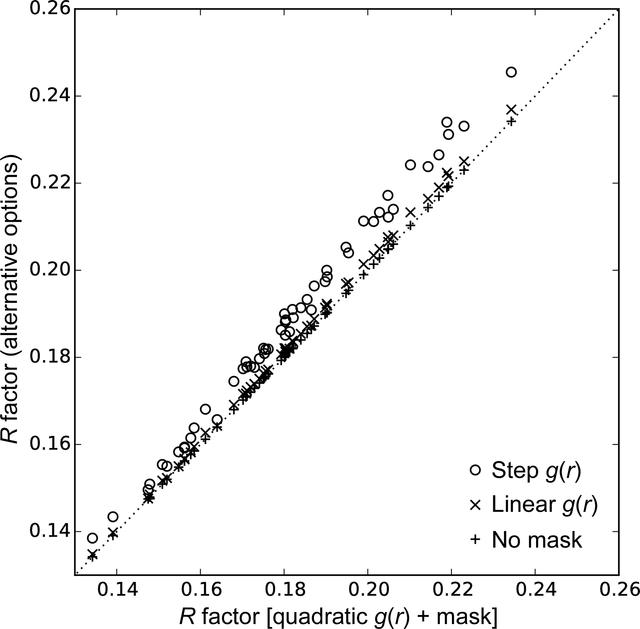disable zoom     view article Figure 1 Comparison of the refinement R factor with different radial functions and masking. The x axis is the R factor using the preferred approach of a quadratic form for g(r) and masking the unmodelled regions. The y axis shows the R factor using three alternative approaches: `o' for a step function g(r), `×' for a linear g(r) and `+' for a quadratic g(r) but no masking of unmodelled regions. In each case the R factor is the best value obtained over trials using ten radii for the radial functions. The quadratic g(r) outperforms the other forms; however, masking has little effect.STRUCTURALBIOLOGY
ISSN: 2059-7983
Volume 74| Part 2| February 2018| Pages 125-131
Follow Acta Cryst. D